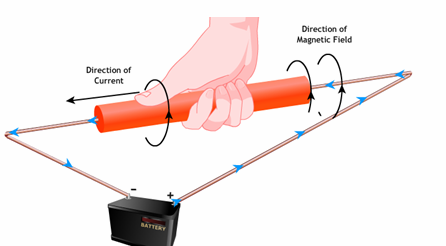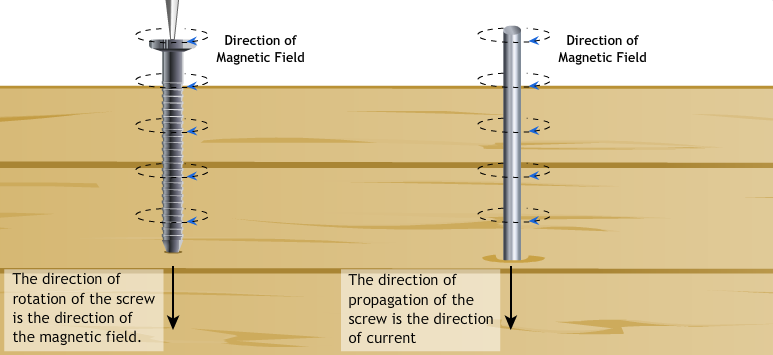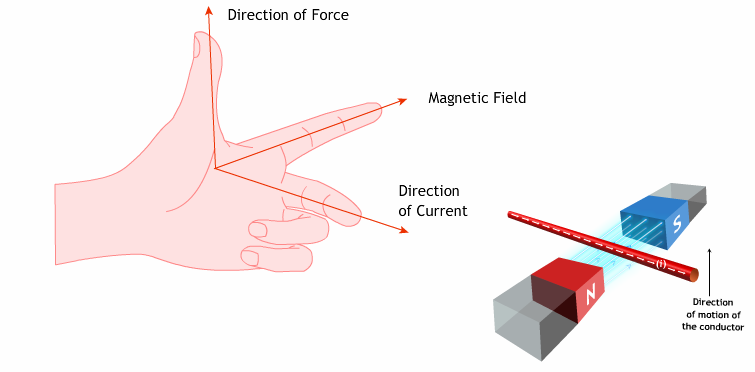Notes On Magnetic Effects of Electric Current - CBSE Class 10 Science
Magnetic effect of electric current is one of the major effects of electric current in use, without the applications of which we cannot have motors in the existing world. A current carrying conductor creates a magnetic field around it, which can be comprehended by using magnetic lines of force or magnetic field lines. The nature of the magnetic field lines around a straight current carrying conductor is concentric circles with centre at the axis of the conductor. The direction of the magnetic field lines of force around a conductor is given by the Maxwell’s right hand grip rule or the right handed cork screw rule. The strength of the magnetic field created depends on the current through the conductor. If the conductor is in the form of a circular loop, the loop behaves like a magnet. Clock-S rule Cloak-S rule is rule which helps us to find the formation of magnetic South Pole due to electromagnetic induction in a current carrying conducting coil. According to clocks rule if one face of a current carrying conducting coil is placed such that one face of the coil is faced to us and current is moving in the clockwise direction with respect to us then the face of the coil which is faced to us becomes as a magnetic south pole and the other face behaves as the north magnetic pole.   A current carrying conductor in the form of a rectangular loop behaves like a magnet and when suspended in an external magnetic field experiences force. SNOW Rule SNOW rule states that if the current is flowing in an electric circuit from South to North direction and a magnetic compass is placed Over the conducting wire, the needle of the compass deflects in the direction of west. Right hand thumb ruleRight hand thumb rule states that if we hold the conductor in the right hand such that the thumb points in the direction of electric current, then the direction in which the fingers curl gives the direction of the magnetic field If we point thumb down wards in the direction of the current, the magnetic field would be represented by the curled fingers as the circles around the conductor.So, if it is viewed from the above plane this field lines will be clockwise circles, but the direction of the magnetic field at any point on this circular magnetic lines is in the direction of the tangent drawn to the circular magnetic lines at the desired points. Maxwell’s cork-screw rule:Maxwell' cork screw rule is also known as maxwell's right hand thumb ruleIf the head of a cork-Screw is rotated such that the tip of the screw advances in the direction of electric current, then the direction of rotation of the head of the screw represents the direction of the magnetic field aroundthe conductor. A magnetic field caused by a current-carrying conductor consists of sets of concentric lines of force. The direction of the magnetic field lines depends on the direction of the current passed through the conductor.   Electromagnet An electromagnet is a magnet made up of a coil of insulated wire wrapped around a soft iron core that is magnetised only when current flows through the wire. Solenoid The solenoid is an electro magnet which is a long cylindrical coil of wire consisting of a large number of turns bound together very tightly. Note:  The length of the coil should be longer than its diameter. (Or) Solenoid is a coil of a number of turns of insulated copper wire closely wrapped in shape of a cylinder. Magnetic field around a current carrying solenoid is as shown in the figure.Theses appear to be similar to that of a bar magnet. One end of the solenoid behaves like North Pole and the other end behaves like the South Pole. Magnetic field lines inside the solenoid are in the form of parallel straight lines. This means that the field is same at all the points inside the solenoid.   When soft iron rod is placed inside the solenoid, it behaves like an electro magnet. The use of soft iron as core in the solenoid produces the strongest magnetism. A solenoid consists of an insulated conducting wire wound on a cylindrical tube made of plastic or cardboard.  Fleming’s left hand ruleIf the forefinger, middle finger and thumb of the left hand are stretched such that they are at right angles to each other, then:The forefinger gives the direction of the magnetic field.        •  $\frac{\text{}}{\text{}}$The middle finger points in the direction of the current.        •  The thumb gives the direction of the force acting on the current-carrying conductor placed in the external magnetic field.         •  An electric motor converts electrical energy into mechanical energy using the magnetic effect of electricity. Electric motor The direction of the force is given by Fleming’s left hand rule. This gives the basis for an electric motor. An electric motor essentially consists of a coil as an armature, a split ring commutator for changing the direction of the current in the coil. There are two brushes linked with the split rings that maintain the contact with the armature for the current flow. Electric motor converts electrical energy to mechanical energy.   A number of such loops form a coil and the coil is termed solenoid. If there is a soft iron core in the solenoid, it behaves like a magnet as long as there is current through the coil. Thus it is an electromagnet.   When an electric current passes through a conductor, a magnetic field is created around the conductor. This phenomenon is known as the magnetic effect of electricity.   A magnetic field is the extent of space surrounding a magnet where the magnet’s effect can be felt. Magnetic field lines represent the lines of action of the force acting on a unit North Pole placed in a magnetic field.

#### Summary

Magnetic effect of electric current is one of the major effects of electric current in use, without the applications of which we cannot have motors in the existing world. A current carrying conductor creates a magnetic field around it, which can be comprehended by using magnetic lines of force or magnetic field lines. The nature of the magnetic field lines around a straight current carrying conductor is concentric circles with centre at the axis of the conductor. The direction of the magnetic field lines of force around a conductor is given by the Maxwell’s right hand grip rule or the right handed cork screw rule. The strength of the magnetic field created depends on the current through the conductor. If the conductor is in the form of a circular loop, the loop behaves like a magnet. Clock-S rule Cloak-S rule is rule which helps us to find the formation of magnetic South Pole due to electromagnetic induction in a current carrying conducting coil. According to clocks rule if one face of a current carrying conducting coil is placed such that one face of the coil is faced to us and current is moving in the clockwise direction with respect to us then the face of the coil which is faced to us becomes as a magnetic south pole and the other face behaves as the north magnetic pole.   A current carrying conductor in the form of a rectangular loop behaves like a magnet and when suspended in an external magnetic field experiences force. SNOW Rule SNOW rule states that if the current is flowing in an electric circuit from South to North direction and a magnetic compass is placed Over the conducting wire, the needle of the compass deflects in the direction of west. Right hand thumb ruleRight hand thumb rule states that if we hold the conductor in the right hand such that the thumb points in the direction of electric current, then the direction in which the fingers curl gives the direction of the magnetic field If we point thumb down wards in the direction of the current, the magnetic field would be represented by the curled fingers as the circles around the conductor.So, if it is viewed from the above plane this field lines will be clockwise circles, but the direction of the magnetic field at any point on this circular magnetic lines is in the direction of the tangent drawn to the circular magnetic lines at the desired points. Maxwell’s cork-screw rule:Maxwell' cork screw rule is also known as maxwell's right hand thumb ruleIf the head of a cork-Screw is rotated such that the tip of the screw advances in the direction of electric current, then the direction of rotation of the head of the screw represents the direction of the magnetic field aroundthe conductor. A magnetic field caused by a current-carrying conductor consists of sets of concentric lines of force. The direction of the magnetic field lines depends on the direction of the current passed through the conductor.   Electromagnet An electromagnet is a magnet made up of a coil of insulated wire wrapped around a soft iron core that is magnetised only when current flows through the wire. Solenoid The solenoid is an electro magnet which is a long cylindrical coil of wire consisting of a large number of turns bound together very tightly. Note:  The length of the coil should be longer than its diameter. (Or) Solenoid is a coil of a number of turns of insulated copper wire closely wrapped in shape of a cylinder. Magnetic field around a current carrying solenoid is as shown in the figure.Theses appear to be similar to that of a bar magnet. One end of the solenoid behaves like North Pole and the other end behaves like the South Pole. Magnetic field lines inside the solenoid are in the form of parallel straight lines. This means that the field is same at all the points inside the solenoid.   When soft iron rod is placed inside the solenoid, it behaves like an electro magnet. The use of soft iron as core in the solenoid produces the strongest magnetism. A solenoid consists of an insulated conducting wire wound on a cylindrical tube made of plastic or cardboard.  Fleming’s left hand ruleIf the forefinger, middle finger and thumb of the left hand are stretched such that they are at right angles to each other, then:The forefinger gives the direction of the magnetic field.        •  $\frac{\text{}}{\text{}}$The middle finger points in the direction of the current.        •  The thumb gives the direction of the force acting on the current-carrying conductor placed in the external magnetic field.         •  An electric motor converts electrical energy into mechanical energy using the magnetic effect of electricity. Electric motor The direction of the force is given by Fleming’s left hand rule. This gives the basis for an electric motor. An electric motor essentially consists of a coil as an armature, a split ring commutator for changing the direction of the current in the coil. There are two brushes linked with the split rings that maintain the contact with the armature for the current flow. Electric motor converts electrical energy to mechanical energy.   A number of such loops form a coil and the coil is termed solenoid. If there is a soft iron core in the solenoid, it behaves like a magnet as long as there is current through the coil. Thus it is an electromagnet.   When an electric current passes through a conductor, a magnetic field is created around the conductor. This phenomenon is known as the magnetic effect of electricity.   A magnetic field is the extent of space surrounding a magnet where the magnet’s effect can be felt. Magnetic field lines represent the lines of action of the force acting on a unit North Pole placed in a magnetic field.

#### Activities

 Activity 1 childrensuniversity.manchester.ac.uk has developed an interactive online simulation which explains how electricity is generated from various sources, using the principle of a generator. In this one gets to build a wind turbine by assembling the components given. An interactive quiz is given at the end to test the understanding of the topic covered. Go to Acivity Activity 2 learnthings.co.za has created an interactive activity which enables one to understand the concept of electricity and magnetism. In this activity one can see the shape of a magnetic field around a single magnet.as well as two magnets. It enables to understand how the fields interact when the poles are repelling or attracting. Also the magnetic field around straight and coiled conductors can be manipulated by changing the direction of the current. Go to Activity